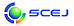\$B2=3X9)3X2qBh(B53\$B2s=)5(Bg2q(B 2022.9.14(\$B?e(B) - 9.16(\$B6b(B) \$B?.=#Bg3X(B \$BD9Ln(B(\$B9)3X(B)\$B%-%c%s%Q%9(B / \$B%*%s%i%\$%s(B English page
Last Update: 2022-05-28 13:48:46

## \$BDs=P:Q\$_%G!<%?\$N1\Mw(B

\$B\$3\$3\$+\$iDs=P:Q\$_\$N9V1i?=9~=q!&9V1iMW;]!&%]%9%?!<(B PDF \$B%U%!%\$%k\$r8+\$k\$3\$H\$,\$G\$-\$^\$9!# \$B"(!V9V1iHV9f%F%9%HDI5-HG!W\$rA*Br\$9\$k\$3\$H\$G!"9V1iHV9f\$H4{B8J8;z\$H\$,=E\$J\$i\$J\$\$\$+\$I\$&\$+\$r3NG'\$G\$-\$k\$h\$&\$K\$J\$j\$^\$7\$?!#(B

\$B \$B9V1i?=9~=q(B \$B9V1iMW;](B(\$B9V1iHV9fDI5-A0(B) \$B9V1iMW;](B(\$B9V1iHV9f%F%9%HDI5-HG(B) \$B%]%9%?!<(B(\$B9V1iHV9fDI5-A0(B) \$B%]%9%?!<(B(\$B9V1iHV9f%F%9%HDI5-HG(B)
 \$B]%G!<%?\$rA*Br\$7\$F\$+\$i!"(B [ OK ] \$B\$r%/%j%C%/\$7\$F\$/\$@\$5\$\$!#(B

\$B%Q%9%o!<%I\$,J,\$+\$i\$J\$\$J}\$O(B\$B\$3\$A\$i(B

This page was generated byeasp 2.47; mknote 2.45 (C)1999-2020 kawase